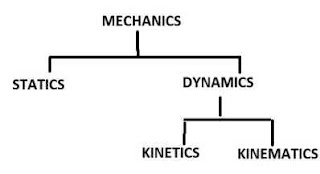What Is Difference Between Statics and Dynamics and Kinematics And Kinetics

In this mechanical engineering lecture today we learned engineering mechanics topic What Is Difference Between Statics and Dynamics and Kinematics And Kinetics .

What Is Difference Between Statics and Dynamics and Kinematics And KineticsWhat Is Difference Between Statics and Dynamics and Kinematics And Kinetics

Engineering mechanics

Engineering mechanics is a branch of engineering science, which deals about a principle of mechanics along with there application.

Engineering mechanics is also branch of physical science that deals with energy and loads and their effects on bodies.

Engineering mechanics is sub- divided into following two parts as follows

1. Statics
2. Dynamics

Statics is branch of engineering mechanics which is deals about the force (load) and their effects when body at rest position.

Statics is deals with the analysis of loads and their effect on system when it's acceleration is zero or relative motion is zero.

Statics is mainly deals with the load acting on stationary bodies that means at equilibrium.

Statics is deals with the forces acting on stationary body. All the force acting on bodies is equilibrium.
( i.e. summation of forces along x-axis and y-axis is zero. ; movement about a point is Zero )

2. Dynamics

Dynamics is branch of engineering mechanics which is deals about force and their effects when body is motion.

Dynamics is study of force when bodies is moving.

Dynamics is sub divided into following two parts as follows

A. Kinetics
B. Kinematics

A. Kinetics

Kinetics is a branch of Dynamics,  which deals with the bodies in motion due to application of force.

Kinetics is used for to learn about the properties such as displacement, acceleration, velocity and their variation with time.

kinetics is study of movement with considering reason of movement.

Example of kinetics
- study of motion of transmission pulley, gears etc.

B. Kinematics

Kinematics is a branch of Dynamics, which deals with the bodies in motion without considering force for body motion.

Kinematics is concerned about the relationship between the motion of the system and causes of motion.

kinematics is study of movement without knowing the reason of movement.

In this mechanical engineering lecture today we learned engineering mechanics topic What Is Difference Between Statics and Dynamics and Kinematics And Kinetics . If you are any queri then comment

Previous
Next Post »